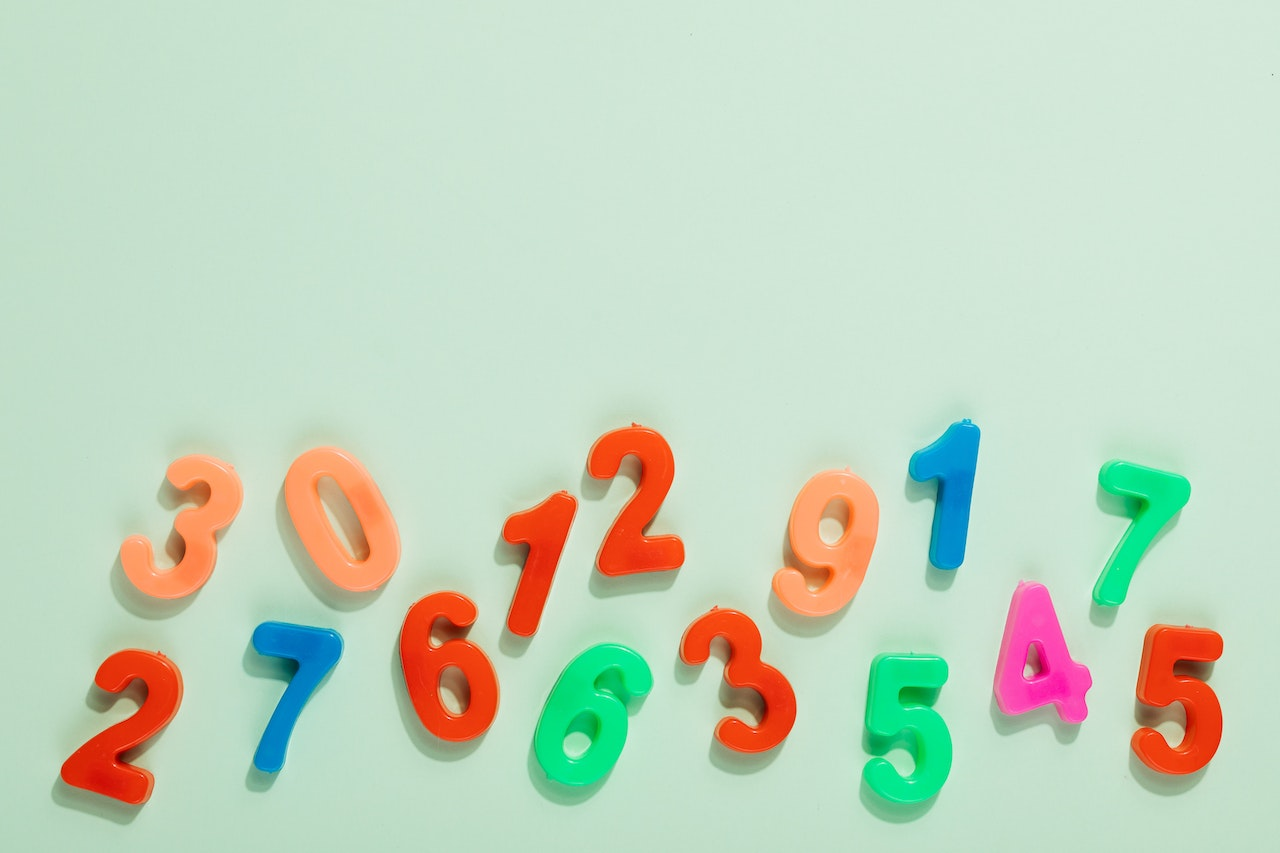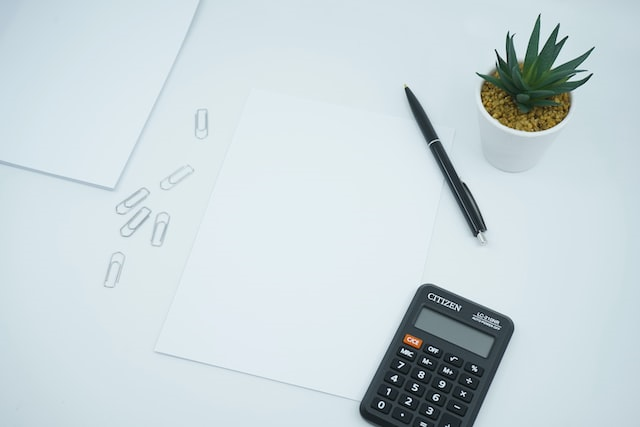The Mode in Math - Definition And How to Calculate it
Nov 29, 2022
Mathematics - Other

## The Mode in Math - Definition And How to Calculate it

Statistics and data come up in our daily lives a lot. Whether reading or watching the news, figuring out probabilities yourself, or simply solving a math problem, you need to understand how different ways of sorting through data and identifying key patterns can be done.

And that's where mode in math can be incredibly useful. Together with the mean and median, the mode is one of the three main measures of central tendency, which indicates the value at the center of a data set.

There are countless applications for mode in math, statistics, and beyond, so it's essential to clearly understand how it works and how you can get it. And the good news is that mode is relatively simple to understand, especially once you familiarize yourself with what it means and how to approach it.

In this guide, let's look at what mode is, how to use it, and how to calculate mode in math using different methods.

### What is the Mode in Math?The mode in math describes the most frequent value in a provided data set. No matter how much data there is or how it's spread out, the mode only looks at the most common value in the entire list, which can be helpful in situations where you need to identify which value is best represented.

There are many types of modes that can occur, which depend on how many values have the highest frequency. If there's just one value (or number) that is the most frequent, that will make the mode unimodal. If the number of values with matching frequencies is higher than one, that will change the mode type accordingly.

One way that mode in math differs from the median and the mean mathematical formulas is that it can be used on something other than numbers. For instance, it could measure the most common color of leaves, knitting patterns, Greek letters, mathematical symbols, or almost any other list of values where the total number of different values can be calculated and compared.

The mode in math is very useful when you want to quickly identify the frequency distribution, evaluate qualitative data, and use values other than numbers. However, it can't be used when there are no repeats in a data set and isn't very useful when there's a small number of values. It also doesn't really provide much help when trying to take into account more extreme values.

## Types of Modes

As mentioned before, the type of mode you get will depend on how many in the list of values have the same highest frequency. So here are the types of modes you can encounter:

• A unimodal mode is a collection of data where only one mode has the highest frequency. For example, if you were to have a group of numbers 2, 2, 4, 7, 7, 3, 9, 7, your mode would be seven since it repeats three times while others are only repeated twice at most.

• A bimodal mode contains two modes of the same frequency. Since they both repeat the same number of times, no one of the values can be called the modal value, making both of them modal, which results in a bimodal mode. For example, you could have a set of numbers 3, 3, 6, 4, 5, 5, 9, 8. The two bimodal values would be three and five because they both repeat two times, while the others repeat only once.

• A trimodal mode is a collection of data where three modes are identified. In this type of mode, three data types have the same frequency in a data set, following the same principle as in the examples above.

• Finally, a multimodal mode is a mode that has four or more data values of the same frequency. When you have four or more modes, the mode's usefulness significantly diminishes since it doesn't provide you with valuable insights.

## Mode vs. Mean vs. Median

To better understand what the mode in math represents, it's a good idea to compare it with the other main measures of central tendency. The mean and the median are equally useful in different situations, so by having a basic grasp of what they help calculate, you will gain a better understanding of what the mode's function is as well.

### The Mean

The mean is the simplest way to measure central tendency, providing an exact average amount for a data set. For example, if you have a set of numbers, you could calculate the mean by adding them all together and dividing them by the total amount of numbers in the data set. Then, you would get the average amount in the data set when taking into account each value that was in it.

### The Median

The median is used to calculate the middle number of a data set. First, you arrange the numbers from smallest to largest, and then the number that is in the very middle of the data set is the median value. When you have an uneven set of numbers, you can calculate without extra steps since the middle number will always be single. But if you have an even number, you will have to add up the two middle numbers and divide them by two, which will give you the median number of the entire data set.

## How to Calculate the ModeFinding the mode can be relatively simple since it doesn't require you to add up or subtract any numbers (or other values) in a data set. Instead, you simply have to arrange the values in a way that makes it easy to calculate how many times each value was used.

Then, the value or values that were used the most often will be the mode in that data set. If there's a single value, that makes it a unimodal mode, and if there's more than one value with the highest frequency, that makes it a bimodal, trimodal, or multimodal mode, depending on the number of values.

If you don't want to manually calculate the mode in math with a pen and paper, some calculators also have a mode function to make the process easier. Using it, you can input each number from your data set and then allow it to calculate. In some calculators, you may need to separate the numbers with a comma for them to work.

On your computer, Excel is probably the most convenient additional tool for getting the correct calculation of the mode in math:

Add each number in your data set into separate cells in a column.

1. Click the "sort" button and select "Sort A to Z" or the opposite. At this point, you should choose a cell where you want the mode to appear.

2. Type in the function "MODE:(beginning cell label/ending cell label)," inputting the labels of the cells you want to be included in the data set when calculating the mode for math.

3. When you work with relatively small data sets, you can usually get by with calculating on paper because there are not a lot of values to go through. But with longer data sets, it will usually be more practical to paste the entire set into a tool like Excel and have it quickly arrange and calculate the mode in math for you.

## Bottom Line

Figuring out how to calculate the mode in math can seem like a daunting task at first. Looking at the data set and not knowing where to start can discourage even the most motivated student from learning. The good news is that, as you saw above, calculating the mode is actually relatively straightforward and even intuitive once you know how it works.

If you still want some help with figuring out the mode in math or other measures of central tendency, we are here to help you with any questions you might have.

View Available Mathematics Tutors24houranswers.com Parker Paradigms, Inc 5 Penn PLaza, 23rd Floor New York, NY 10001
SUBJECTS COVERED